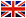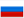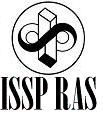Basics of the experimental techniques

E.V. Deviatov, DrS

1. Introduction. Applied and fundamental physics.
2. Units of physical quantities, systems of units, standards.
3. Methods and strategy of measurements. Theory of errors.
4. Characteristics of the experimental setups. Description of the measuring systems by differential equations.
5. Backward influence of the measuring system on the experimental object: matching. Noise, stray pick-up.
6. Structure of the measuring systems. Sensors.
7. Structure of the measuring systems. Amplification. Lock-in amplifier.
8. Structure of the measuring systems. Digital-analog conversion. Analog-digital conversion.
9. Interface between the computer and the measuring system. IEEE-488 standard.
10. Cryogenic liquids. Cryogenic technique.
11. Vacuum technique. Pumps.
12. Magnetic field.
13. Lasers.
14. High-frequency generators and measuring systems.Agarkov D.A. • Tel: +7(916)7584930 • email: agarkov@issp.ac.ru